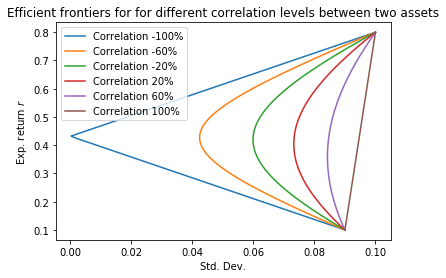# Exploring the efficient frontier

## Correlation & the Efficient Frontier¶

We show the importance of correlation in creating an investment portfolio and how to generate an efficient frontier based on two assets. The same problem is scalable to multiple assets.

In :
import numpy as np
import matplotlib.pyplot as plt


We randomly select returns and standard deviations for assets a and b.

In :
r_a = 0.10
r_b = 0.8
stdev_a = 0.09
stdev_b = 0.10


We use different correlations of ranging from -100% (negatively correlated) to 100% (perfectly correlated). We find that the negatively correlated assets result in the largest efficient frontier. Thus this shows that negatively correlated assets result in the greatest risk-adjusted return

In :
for correl in np.linspace(-1,1,6):
X, Y = [], []
for w_a in np.linspace(0,1,100):
w_b = 1 - w_a
r = r_a * w_a + r_b * w_b
var = w_a**2 * stdev_a**2 + w_b**2 * stdev_b**2 + 2*w_a*w_b*stdev_a*stdev_b*correl
stdev = np.sqrt(var)
X.append(stdev)
Y.append(r)
plt.plot(X,Y,label='Correlation %0.0f%%' % (100*correl))

plt.xlabel('Std. Dev.'), plt.ylabel('Exp. return $r$')
plt.title('Efficient frontiers for for different correlation levels between two assets')
plt.legend();In [ ]: